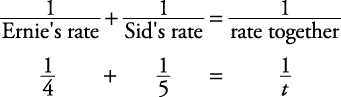## Work Problems

##### Example 1

Ernie can plow a field alone in four hours. It takes Sid five hours to plow the same field alone. If they work together (and each has a plow), how long will it take to plow the field?

First, circle what you must find— how long …together. Work problems of this nature may be solved by using the following equation.Therefore,Finding a common denominatorCross multiplyingTherefore, it will take themhours working together.